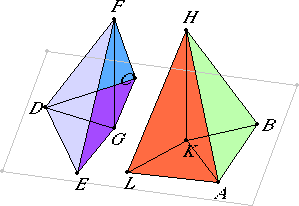# Proposition 26

To construct a solid angle equal to a given solid angle on a given straight line at a given point on it.

Let A be the given point on the given straight line AB, and let the angle at D be the given solid angle contained by the angles EDC, EDF, and FDC.

It is required to construct at the point A on the straight line AB a solid angle equal to the solid angle at D.

XI.11

Take a point F at random on DF, draw FG from F perpendicular to the plane through ED and DC, and let it meet the plane at G. Join DG.I.23

At the point A on the straight line AB construct the angle BAL equal to the angle EDC, and construct the angle BAK equal to the angle EDG.

XI.12

Make AK equal to DG. Set KH up from the point K at right angles to the plane through BA and AL. Make KH equal to GF, and join HA.

I say that the solid angle at A contained by the angles BAL, BAH, and HAL equals the solid angle at D contained by the angles EDC, EDF, and FDC.

Cut AB and DE off equal to one another, and join HB, KB, FE, and GE.

XI.Def.3

Then, since FG is at right angles to the plane of reference, therefore it is also at right angles with all the straight lines which meet it and are in the plane of reference. Therefore each of the angles FGD and FGE is right. For the same reason each of the angles HKA and HKB is also right.

I.4

And, since the two sides KA and AB equal the two sides GD and DE respectively, and they contain equal angles, therefore the base KB equals the base GE. But KH also equals GF, and they contain right angles, therefore HB also equals FE. Again, since the two sides AK and KH equal the two sides DG and GF, and they contain right angles, therefore the base AH equals the base FD.

I.8

But AB also equals DE, therefore the two sides HA and AB are equal to the two sides DF and DE. And the base HB is equal to the base FE, therefore the angle BAH equals the angle EDF. For the same reason the angle HAL also equals the angle FDC.

And the angle BAL also equals the angle EDC. Therefore at the point A on the straight line AB a solid angle has been constructed equal to the given solid angle at D.

Q.E.F.

## Guide

This construction is used in the next one to construct similar parallelepipeds.